Open in App
Not now

# ∈-NFA of Regular Language L = bc(ab+c)*a+

• Last Updated : 05 Mar, 2021

Finite Automata can be classified into three types-

1. DFA
2. NFA
3. ∈-NFA.

The only difference between ∈-NFA and NFA is that ∈-NFA has a different transition function than regular NFA. ∈ represents empty inputs. ∈-NFA shows that an automaton can change its state without an input, i.e. even if the input is null the automaton can change its state.  Here is the formal definition of ∈-NFA.

```∈-NFA is defined in 5 tuple representation {Q, q0, Σ, δ, F} where
Q is the set of all states,
q0 is the initial state,
Σ is the set of input symbols,
δ is the transition function which is δ:Q × (Σ∪∈)⇢2Q and
F is the set of final states.```

There are a few special rules for the construction of ∈-NFA.

Simple rules for construction of ∈-NFA –

1. ∈-NFA for a+ :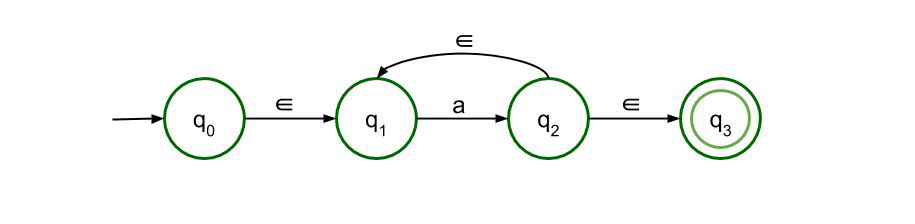The first rule states that at least one ‘a’ needs to be in the expression to be acceptable when it has ‘a+’. ∈ shows the lack of other symbols in the expression which will not make it invalid. However, there is feedback from state q2 to q1 which shows that there can be more than one ‘a’.

2. ∈-NFA for a* :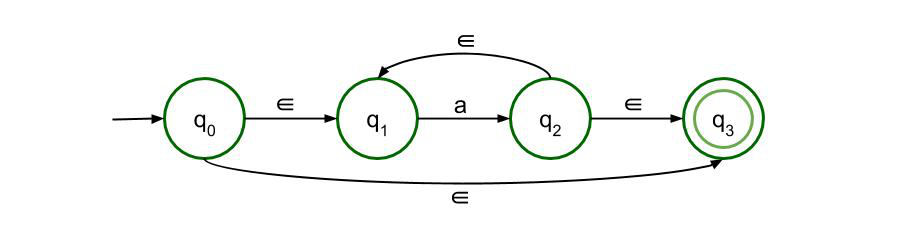The second rule states that ‘a*’ means that there can be 0 or n number of ‘a’s in the expression. The structure of a+ has been modified a bit by adding a loop that goes straight from q0 to the final state which means the expression can have nothing at all and still be acceptable.

3. ∈-NFA for a+b :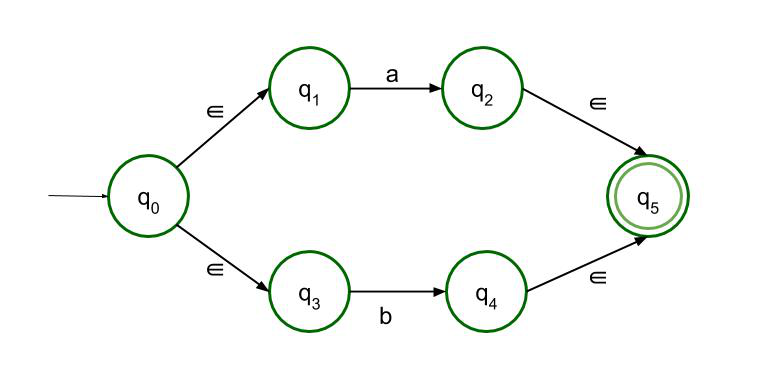The third rule a+b works like the OR logic. It means the expression can have either a or b, and it will be acceptable. So there are two paths, both of which lead to the final state.

4. ∈-NFA for ab :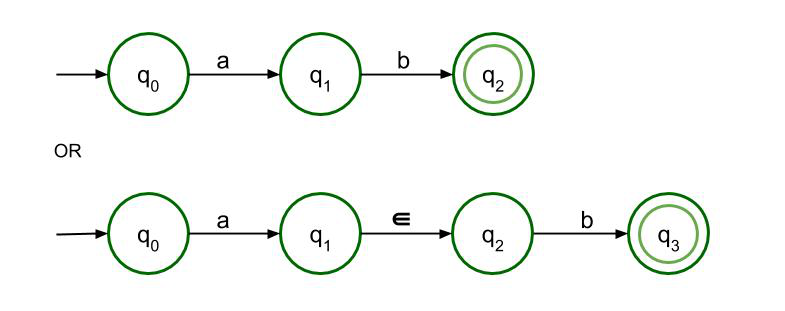The fourth rule is the concatenation rule which states that a must be followed by b. Only then it can reach the final state. Both structures are allowed here but as it is ∈-NFA so the second structure is recommended.

5. ∈-NFA for L = bc(ab+c)*a+ :
Following the above-mentioned rules, ∈-NFA of Regular Language L = bc(ab+c)*a+ is to be constructed.
L = bc(ab+c)*a+ can be divided into 3 parts-

• The first part is ‘bc’ which can be drawn using the concatenation rule, i.e. the fourth rule by swapping a and b with b and c in the original rule, and we consider the extra ∈ here as it is an epsilon NFA.
• The second part is ‘(ab+c)*’ which is a combination of three rules in itself. If we consider ab+c as a unit then (ab+c)* can be drawn with the help of the second rule, a*. Now, ab+c is connected by ‘+’ so we can draw it with the help of the third rule. Since it is an OR connection, so there will be two paths, one having ab and another having c. ‘ab’ can be drawn with the help of the concatenation rule. Below is its ∈-NFA.
• The third part is ‘a+’ which is the first rule itself. Now, all three parts are connected to each other using the concatenation rule.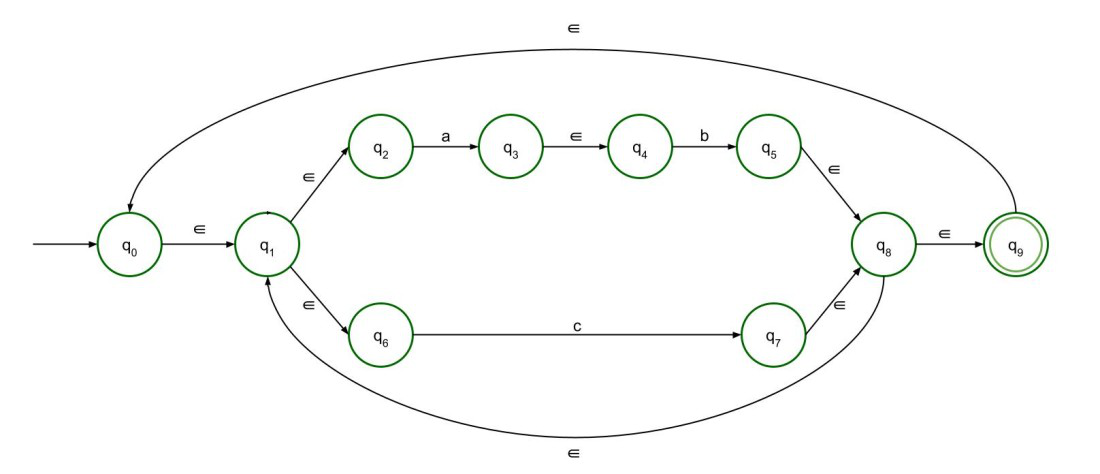The Final ∈-NFA will be –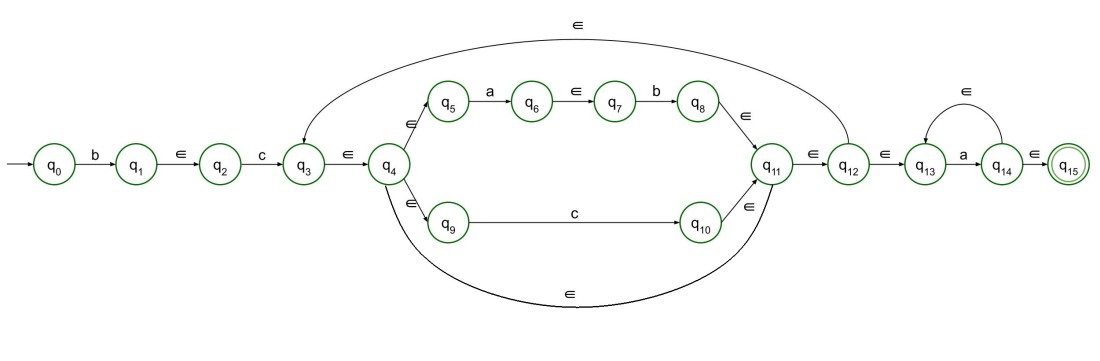My Personal Notes arrow_drop_up